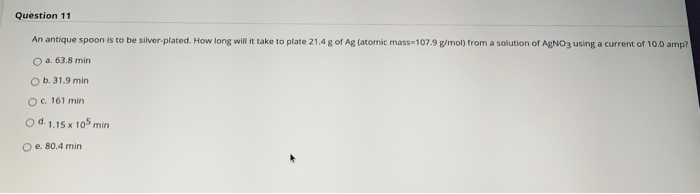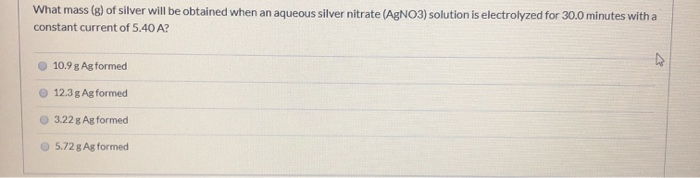Question

A spoon was silver-plated electrolytically in a AgNO3 solution. If 0.750 g of Ag was deposited on the spoon at a constant current of 20.0 mA, how long (in hours) did the electrolysis take? (1 mA = 1x10–3 A)

11.9 hr

9.32 hr

5.92 hr

8.76 hr

10.1 hr

9.32 hr

Explanation

Ag+(aq) + e -------> Ag(s)

1mole of electrons is required to deposite 1mole of Ag+

Number of moles of Ag+ = 0.750g /107.87g/mol = 0.006953mol

number of moles of electrons required = 0.006953mol

Number of Columbs required = 0.006953mol × 96485C/mol = 670.86C

20.0mA = 0.0200A

1Ambs = 1Coloumb per second

0.0200A = 0.0200Couloumbs per second

Therefore

Time required for electrolysis = 670.86C/0.0200C/s = 33543s = 9.32 hr

#### Earn Coins

Coins can be redeemed for fabulous gifts.

Similar Homework Help Questions
• ### Question 11 An antique spoon is to be silver-plated. How long will it take to plate...Question 11 An antique spoon is to be silver-plated. How long will it take to plate 21.4 g of Ag (atomic mass=107.9 g/mol) from a solution of AgNO3 using a current of 10.0 amp? a. 63.8 min b. 31.9 min O c. 161 min Od 1.15 x 105 min O e. 80.4 min Moving to another question will save this response. Question 12 A 25.0 ml sample of 0.20 M Formic Acid (HCO2H, aq) is titrated with 0.10 M KOH(aq)....

• ### What mass (g) of silver will be obtained when an aqueous silver nitrate (AgNO3) solution is...What mass (g) of silver will be obtained when an aqueous silver nitrate (AgNO3) solution is electrolyzed for 30.0 minutes with a constant current of 5.40 A? 10.9 g Ag formed ♡ 12.3 g Ag formed 3.22 g Ag formed 5.72 g Ag formed